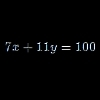# Search by Topic

#### Resources tagged with Diophantine equations similar to Base Puzzle:

Filter by: Content type:
Age range:
Challenge level:

### There are 15 results

Broad Topics > Algebraic expressions, equations and formulae > Diophantine equations### Whole Numbers Only

##### Age 11 to 14 Challenge Level:

Can you work out how many of each kind of pencil this student bought?### Not a Polite Question

##### Age 11 to 14 Challenge Level:

When asked how old she was, the teacher replied: My age in years is not prime but odd and when reversed and added to my age you have a perfect square...### Hallway Borders

##### Age 11 to 14 Challenge Level:

What are the possible dimensions of a rectangular hallway if the number of tiles around the perimeter is exactly half the total number of tiles?### In Particular

##### Age 14 to 16 Challenge Level:

Can you find formulas giving all the solutions to 7x + 11y = 100 where x and y are integers?### Fibs

##### Age 11 to 14 Challenge Level:

The well known Fibonacci sequence is 1 ,1, 2, 3, 5, 8, 13, 21.... How many Fibonacci sequences can you find containing the number 196 as one of the terms?### Upsetting Pitagoras

##### Age 14 to 18 Challenge Level:

Find the smallest integer solution to the equation 1/x^2 + 1/y^2 = 1/z^2### Rudolff's Problem

##### Age 14 to 16 Challenge Level:

A group of 20 people pay a total of £20 to see an exhibition. The admission price is £3 for men, £2 for women and 50p for children. How many men, women and children are there in the group?### Our Ages

##### Age 14 to 16 Challenge Level:

I am exactly n times my daughter's age. In m years I shall be ... How old am I?### Coffee

##### Age 14 to 16 Challenge Level:

To make 11 kilograms of this blend of coffee costs £15 per kilogram. The blend uses more Brazilian, Kenyan and Mocha coffee... How many kilograms of each type of coffee are used?### Letter Land

##### Age 11 to 14 Challenge Level:

If: A + C = A; F x D = F; B - G = G; A + H = E; B / H = G; E - G = F and A-H represent the numbers from 0 to 7 Find the values of A, B, C, D, E, F and H.### Solving with Euclid's Algorithm

##### Age 14 to 18 Challenge Level:

A java applet that takes you through the steps needed to solve a Diophantine equation of the form Px+Qy=1 using Euclid's algorithm.### CD Heaven

##### Age 14 to 16 Challenge Level:

All CD Heaven stores were given the same number of a popular CD to sell for £24. In their two week sale each store reduces the price of the CD by 25% ... How many CDs did the store sell at. . . .### Deep Roots

##### Age 14 to 16 Challenge Level:

Find integer solutions to: $\sqrt{a+b\sqrt{x}} + \sqrt{c+d.\sqrt{x}}=1$### Plutarch's Boxes

##### Age 11 to 14 Challenge Level:

According to Plutarch, the Greeks found all the rectangles with integer sides, whose areas are equal to their perimeters. Can you find them? What rectangular boxes, with integer sides, have. . . .### Are You Kidding

##### Age 14 to 16 Challenge Level:

If the altitude of an isosceles triangle is 8 units and the perimeter of the triangle is 32 units.... What is the area of the triangle?